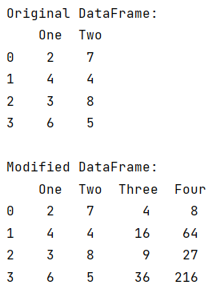# Pandas: Create two new columns in a DataFrame with values calculated from a pre-existing column

Given a Pandas DataFrame, we have to create two new columns in it with values calculated from a pre-existing column.
Submitted by Pranit Sharma, on July 11, 2022

Pandas is a special tool that allows us to perform complex manipulations of data effectively and efficiently. Inside pandas, we mostly deal with a dataset in the form of DataFrame. DataFrames are 2-dimensional data structures in pandas. DataFrames consist of rows, columns, and data.

To create new columns with values from an existing column, we will first define a function to apply certain operations and conditions and finally, we will create a new column with the values returned by the function.

To work with pandas, we need to import pandas package first, below is the syntax:

```import pandas as pd
```

Let us understand with the help of an example,

## Python code to create two new columns in a DataFrame with values calculated from a pre-existing column

```# Importing pandas package
import pandas as pd

# Defining a function
def function(x):
return x**2, x**3

# Creating a dictionary
d= {'One':[2,4,3,6],'Two':[7,4,8,5]}

# Creating a DataFrame
df = pd.DataFrame(d)

# Display original DataFrame
print("Original DataFrame:\n",df,"\n")

# Creating two new columns
df['Three'],df['Four'] = zip(*df['One'].map(function))

# Display modified DataFrame
print("Modified DataFrame:\n",df)
```

Output:Languages: » C » C++ » C++ STL » Java » Data Structure » C#.Net » Android » Kotlin » SQL
Web Technologies: » PHP » Python » JavaScript » CSS » Ajax » Node.js » Web programming/HTML
Solved programs: » C » C++ » DS » Java » C#
Aptitude que. & ans.: » C » C++ » Java » DBMS
Interview que. & ans.: » C » Embedded C » Java » SEO » HR
CS Subjects: » CS Basics » O.S. » Networks » DBMS » Embedded Systems » Cloud Computing
» Machine learning » CS Organizations » Linux » DOS
More: » Articles » Puzzles » News/Updates